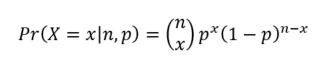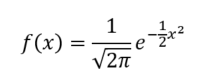# TamStat Distributions

There are two types of distributions: discrete and continuous. Discrete distributions take on a countable number of values which are usually integers starting with 0 or 1. Continuous distributions involve measurement and take on an infinite number of values.

## Discrete Distributions

Discrete distributions are defined by the probability mass function. The parameters of the binomial distribution are n = the number of trials and p the probability of success. The probability mass function for the binomial distribution is:Thus, if we want to find the probability of getting exactly 3 heads in 10 coin tosses, we set n = 10, p = 0.5 and x = 3.

```
10 0.5 binomial 3
0.1171875
```

## Continuous Distributions

Continuous distributions are defined by the density function. For example the standard normal density is:Thus, to obtain the height of the standard normal curve at its center point x=0,

```
normal 0
0.3989422804
```

The density shows the relative likelihood of a particular value from a distribution, but it is not useful, because the probability of an exact value is essentially zero.  The cumulative probability  and interval probabilities are more useful measures; to find these see the section on the probability operator.